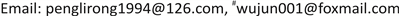1. 引言

2. 数据采集与研究方法2.1. 数据来源

2.2. 研究方法

2.2.1. 最邻近点指数分析

2.2.2. 地理集中指数

G = 100 % * ∑ i = 1 w ( X i / T ) 2

2.2.3. 空间基尼系数

G = − ∑ i = 1 N P i L n P i L n N

2.2.4. 核密度分析

R n ( x ) = 1 / n h ∑ i = 1 n k

2.2.5. 空间自相关分析

I = ∑ i = 1 n ∑ j = 1 n W i j ( X i − X ¯ ) ( X j − X ¯ ) S 2 ∑ i = 1 n ∑ j = 1 n W i j

2.3. 四川省三星及以上星级农家乐的整体分布特征

Statistics of four-star and above-level star farmhouses in four geographical regions in Sichuan Provinc

2.4. 四川省三星及以上星级农家乐的空间分布特征2.4.1. 四川省三星及以上星级农家乐的空间分布特征

2.4.2. 空间自相关分析

Samsung and above star farmhouse space related value
Moran’s IZ值检验得分Z值检验置信区间Z值检验均匀分布区间
0.29850234.49781699%−1.65~1.65

3. 四川省星级农家乐的空间分布的影响因素分析

3.1. 资源禀赋

3.2. 客源市场

3.3. 交通条件

3.4. 地形条件

4. 结论与讨论4.1. 结论

4.2. 讨论